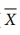Home | | Business Maths 12th Std | Choose the correct Answer

## Chapter: 12th Business Maths and Statistics : Chapter 9 : Applied Statistics

Business Maths and Statistics: Applied Statistics: Multiple choice questions with Answers, Solution - Book back 1 mark questions and answers with solution for Exercise Problems

1. A time series is a set of data recorded

(a) Periodically

(b) Weekly

(c) successive points of time

(d) all the above

2. A time series consists of

(a) Five components

(b) Four components

(c) Three components

(d) Two components

3. The components of a time series which is attached to short term fluctuation is

(a) Secular trend

(b) Seasonal variations

(c) Cyclic variation

(d) Irregular variation

4. Factors responsible for seasonal variations are

(a) Weather

(b) Festivals

(c) Social customs

(d) All the above

5. The additive model of the time series with the components T, S, C and I is

(a) y=T+S+C×I

(b) y=T+S×C×I

(c) y=T+S+C+I

(d) y=T+S×C+I

6. Least square method of fitting a trend is

(a) Most exact

(b) Least exact

(c) Full of subjectivity

(d) Mathematically unsolved

7. The value of ‘b’ in the trend line y=a+bx is

(a) Always positive

(b) Always negative

(c) Either positive or negative

(d) Zero

8. The component of a time series attached to long term variation is trended as

(a) Cyclic variation

(b) Secular variations

(c) Irregular variation

(d) Seasonal variations

9. The seasonal variation means the variations occurring with in

(a) A number of years

(b) within a year

(c) within a month

(d) within a week

10. Another name of consumer’s price index number is:

(a) Whole-sale price index number

(b) Cost of living index

(c) Sensitive

(d) Composite

11. Cost of living at two different cities can be compared with the help of

(a) Consumer price index

(b) Value index

(c) Volume index

(d) Un-weighted index

12. Laspeyre’s index = 110, Paasche’s index = 108, then Fisher’s Ideal index is equal to:

(a) 110

(b) 108

(c) 100

(d) 109

13. Most commonly used index number is:

(a) Volume index number

(b) Value index number

(c) Price index number

(d) Simple index number

14. Consumer price index are obtained by:

(a) Paasche’s formula

(b) Fisher’s ideal formula

(c) Marshall Edgeworth formula

(d) Family budget method formula

15. Which of the following Index number satisfy the time reversal test?

(a)Laspeyre’s Index number

(b) Paasche’s Index number

(c) Fisher Index number

(d) All of them.

16. While computing a weighted index, the current period quantities are used in the:

(a) Laspeyre’s method

(b) Paasche’s method

(c) Marshall Edgeworth method

(d) Fisher’s ideal method

17. The quantities that can be numerically measured can be plotted on a

(a) p - chart

(b) c – chart

(c) x bar chart

(d) np – chart

18. How many causes of variation will affect the quality of a product?

(a) 4

(b) 3

(c) 2

(d) 1

19. Variations due to natural disorder is known as

(a) random cause

(b) non-random cause

(c) human cause

(d) all of them

20. The assignable causes can occur due to

(a) poor raw materials

(b) unskilled labour

(c) faulty machines

(d) all of them

21. A typical control charts consists of

(a) CL, UCL

(b) CL, LCL

(c) CL, LCL, UCL

(d) UCL, LCL

22.chart is a

(a) attribute control chart

(b) variable control chart

(c) neither Attribute nor variable control chart

(d) both Attribute and variable control chart

23. R is calculated using

(a) xmax − xmin

(b) xmin − xmax24. The upper control limit forchart is given byAns: (c)

25. The LCL for R chart is given byAns: (d)Tags : Applied Statistics , 12th Business Maths and Statistics : Chapter 9 : Applied Statistics
Study Material, Lecturing Notes, Assignment, Reference, Wiki description explanation, brief detail
12th Business Maths and Statistics : Chapter 9 : Applied Statistics : Choose the correct Answer | Applied Statistics